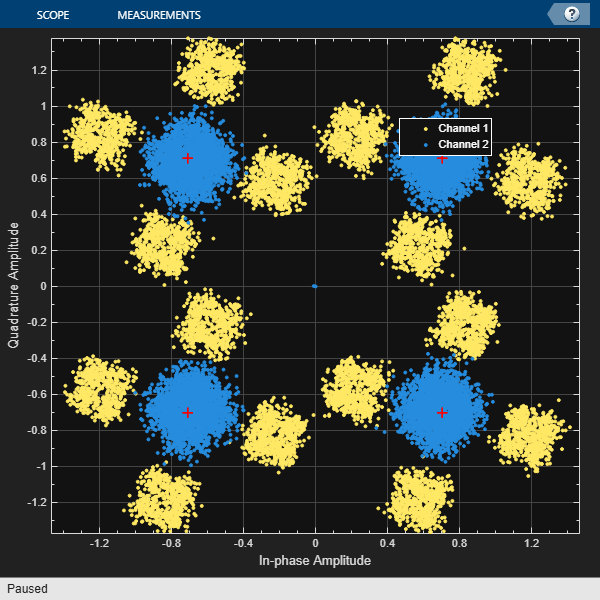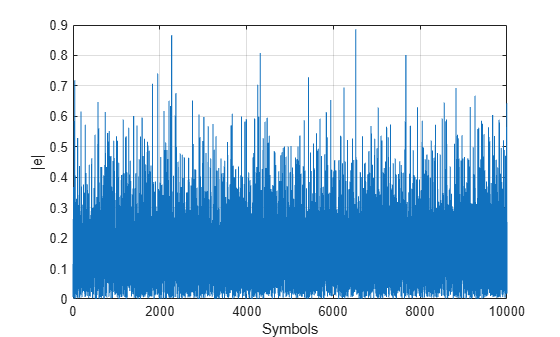# mmseweights

Linear equalizer MMSE tap weights

Since R2019a

## Syntax

``weights = mmseweights(eq,chTaps,EbN0)``

## Description

example

````weights = mmseweights(eq,chTaps,EbN0)` calculated minimum mean squared error (MMSE) solution for the linear equalizer, `eq` System object™ given the channel delay taps, `chTaps`, and signal to noise ratio, `EbN0`.```

## Examples

collapse all

Calculate the minimum mean squared error (MMSE) solution and use the weights for the linear equalizer taps weights.

Initialize simulation variables.

```M = 4; % QPSK numSymbols = 10000; numTrainingSymbols = 1000; chtaps = [1 0.5*exp(1i*pi/6) 0.1*exp(-1i*pi/8)]; EbN0 = 20;```

Generate QPSK modulated symbols. Apply delayed multipath channel filtering and AWGN impairments to the symbols.

```data = randi([0 M-1], numSymbols, 1); tx = pskmod(data, M, pi/4); rx = awgn(filter(chtaps,1,tx),25,'measured');```

Create a linear equalizer System object configured to use CMA algorithm and input the taps weights. Calculate the MMSE weights. Set the initial tap weights to the calculated MMSE weights. Equalize the impaired symbols.

`eq = comm.LinearEqualizer('Algorithm','CMA','AdaptWeights',false,'InitialWeightsSource','Property')`
```eq = comm.LinearEqualizer with properties: Algorithm: 'CMA' NumTaps: 5 StepSize: 0.0100 Constellation: [0.7071 + 0.7071i -0.7071 + 0.7071i -0.7071 - 0.7071i 0.7071 - 0.7071i] InputSamplesPerSymbol: 1 AdaptWeightsSource: 'Property' AdaptWeights: false InitialWeightsSource: 'Property' InitialWeights: [5x1 double] WeightUpdatePeriod: 1 ```
`wgts = mmseweights(eq,chtaps,EbN0)`
```wgts = 5×1 complex 0.0005 - 0.0068i 0.0103 + 0.0117i 0.9694 - 0.0019i -0.3987 + 0.2186i 0.0389 - 0.1756i ```
```eq.InitialWeights = wgts; [y,err,weights] = eq(rx);```

Plot constellation of impaired and equalized symbols.

```constell = comm.ConstellationDiagram('NumInputPorts',2); constell(rx,y)```Plot the equalizer error signal and compute the error vector magnitude of the equalized symbols.

```plot(abs(err)) grid on; xlabel('Symbols'); ylabel('|e|')``````errevm = comm.EVM; evm = errevm(tx,y)```
```evm = 139.0863 ```

Plot equalizer tap weights.

```subplot(3,1,1); stem(real(weights)); ylabel('real(weights)'); xlabel('Tap'); grid on; axis([1 8 -0.5 1]) line([eq.NumTaps+0.5 eq.NumTaps+0.5], [-0.5 1], 'Color', 'r', 'LineWidth', 1) title('Equalizer Tap Weights') subplot(3,1,2); stem(imag(weights)); ylabel('imag(weights)'); xlabel('Tap'); grid on; axis([1 8 -0.5 1]) line([eq.NumTaps+0.5 eq.NumTaps+0.5], [-0.5 1], 'Color', 'r', 'LineWidth', 1) subplot(3,1,3); stem(abs(weights)); ylabel('abs(weights)'); xlabel('Tap'); grid on; axis([1 8 -0.5 1]) line([eq.NumTaps+0.5 eq.NumTaps+0.5], [-0.5 1], 'Color', 'r', 'LineWidth', 1)```## Input Arguments

collapse all

Equalizer object, specified as a `comm.LinearEqualizer` System object.

Channel delay taps, specified as a vector.

Data Types: `double`
Complex Number Support: Yes

Signal to noise ratio of the channel, specified as a scalar.

Data Types: `double`

## Output Arguments

collapse all

Weights for linear equalizer, returned as a vector.

## Version History

Introduced in R2019a